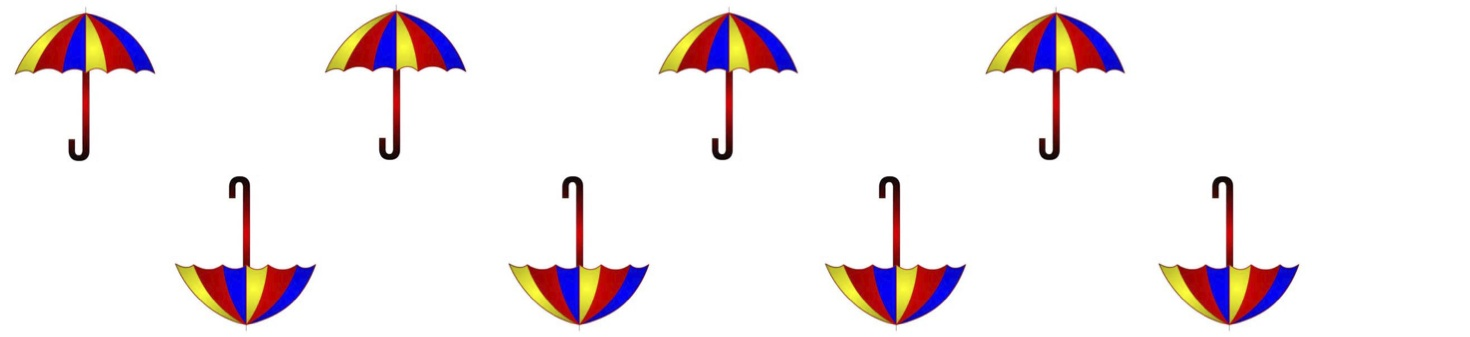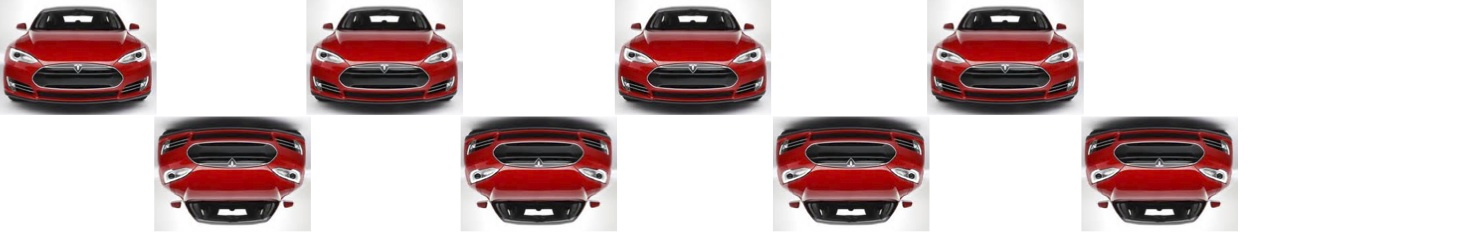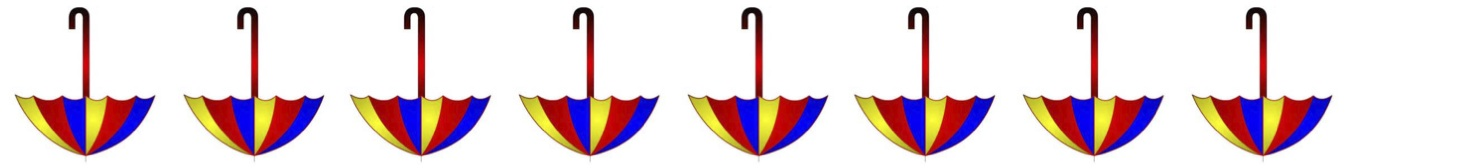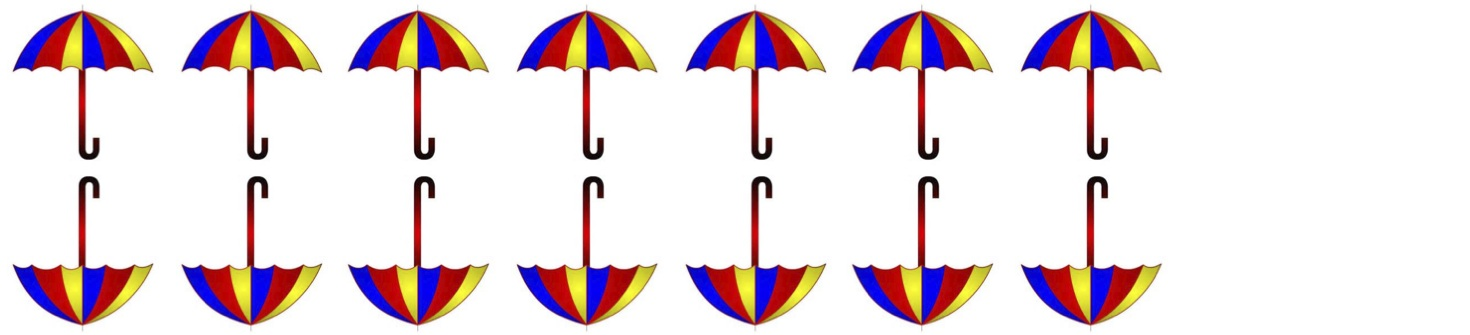Symmetry Quiz
Name: *1. Name the above symmetry of the cars *2. Name the above symmetry of the umbrellas *3. Name the above symmetry of the cars *4. Name the above symmetry of the umbrellas *
5. How many symmetry motions are there in band symmetries *
6. How many symmetry types are there in band symmetries *7. Name the motion in the symmetry above *8. Name the symmetry type above *9. Name the symmetry type above *10. Name the symmetry type above *